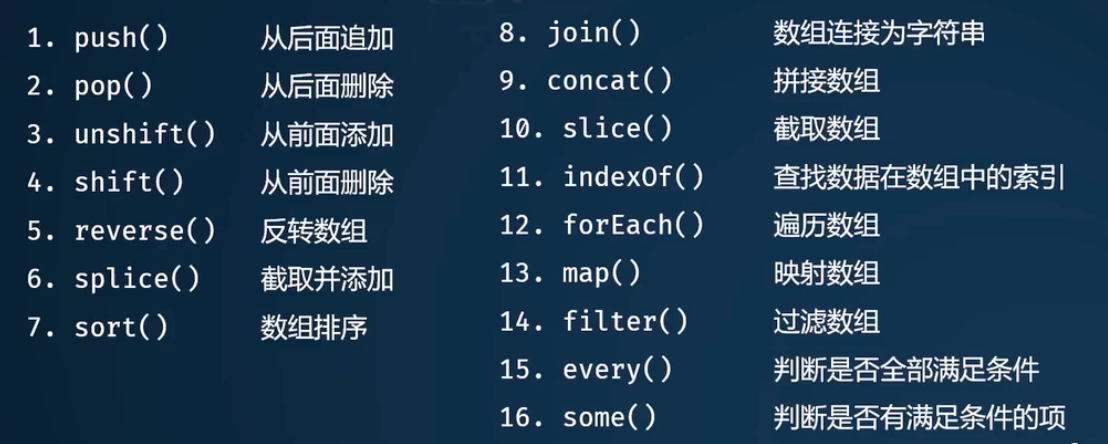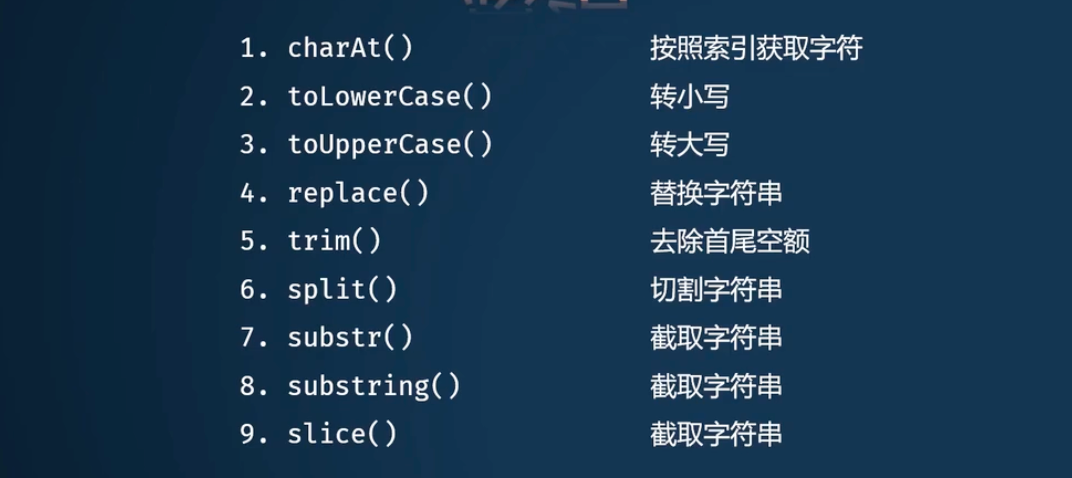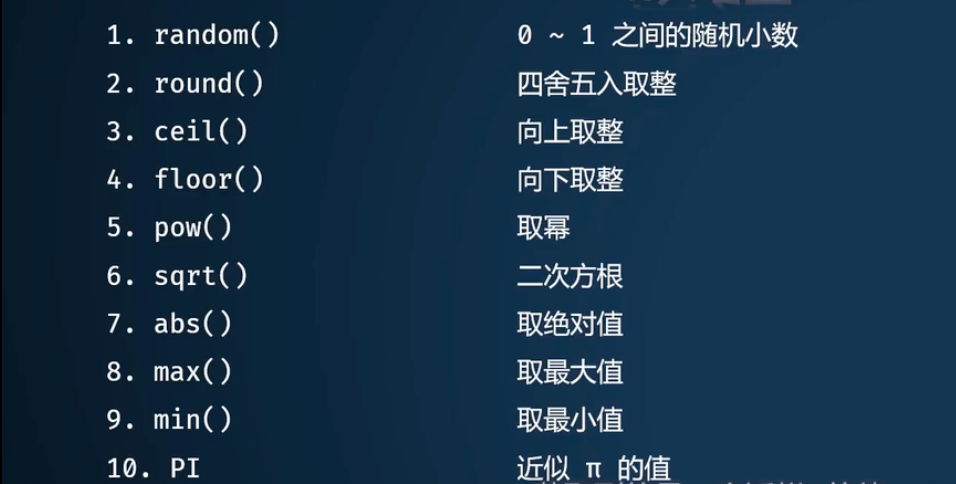# 数组// push 在数组末尾追加数据，返回最新长度
// pop 删除数组最后一个数据，返回删除的数据
var new_lenght = arr.push('new data');
var delete_data = arr.pop();

// unshift 在数组开始追加数据，返回最新长度
// shift 删除数组最前一个数据，返回删除的数据
var new_lenght = arr.unshift("start data");
var delete_data = arr.shift();

// reverse 数组反转
var new_arr = arr.reverse()

// splice 删除 数组中若干数据，并选择是否插入新的数据
// 参数：开始索引（0），多少个（0）， 要插入的数据（没有）
// 以新数组的形式返回被删除的那些数据
var delete_arr = arr.splice(1, 1);  // 索引1开始删除一个数据，无插入
var delete_arr = arr.splice(1, 1, 'new data');  // 索引1开始删除一个数据，并在删除位置插入新数据

// sort 排序，本身返回按位排序的，非自然序列
// 升序：sort(function(a, b){return a-b;});
// 降序：sort(function(a, b){return b-a;});
// 返回排序的数组
var new_arr = arr.sort();

// join 使用连接符，将数组的元素连接成一个字符串
var strs = arr.join('-');

// concat 数据拼接，在前一个数组后面扩展
var new_arr = arr1.concat(arr2);

// slice 切片
// 参数：开始索引（默认0），结束索引（默认数组长度），返回切片数组
var new_arr = arr.slive(1);

// indexOf 查找数据在数组中第一次出现的位置，没有 就是返回-1
// 参数：查找的数据
var data_index = arr.indexOf(data);

// forEach 遍历数组
arr.forEach(function(item, index, arr){
// to do
})

// map 返回新数组
var new_arr = arr.map(function(item, index, arr){
// 映射条件
return item * 10;
})

// filter 过滤数组
var new_arr = arr.filter(function(item, index, arr){
// 过滤条件
return item > 10;
})

// every 判断数组是不是每一项都满足条件，返回一个布尔值
var is_right = arr.every(function(item, index, arr){
// 过滤条件
return item > 10;
})

// some 数组中是不是有某一项满足条件，返回一个布尔值
var is_right = arr.some(function(item, index, arr){
// 过滤条件
return item > 10;
})



# 字符串// charAt 获取对应位置字符，数组越界？
var str = strs.charAt(3);

// toLowerCase 所有大写字母全部转化成小写
// toUpperCase 与之相反
var strs = strs.toLowerCase();

// replace 替换
var strs = strs.replace(',', '.');

// trim 去除首尾空格
var strs = strs.trim()

// split 分割
var strs_arr = strs.split('-')

// 截取字符串 后两个有什么区别
// strs.sbustr(开始索引，多少个)
// strs.shustring(开始索引，结束索引)
// strs.slice(开始索引，结束索引)
var new_strs = strs.sbustr(1, 6);  // 从索引1开始截取6个字符
var new_strs = strs.shustring(1, 6)  // [1, 6)
var new_strs = strs.slice(1, 6)  // [1, 6)



# 数字// random 返回[0, 1)上的一个随机小数，小数点后17位
var num = Math.random();

// round 四舍五入保留整数
// ceil 向上取整
// floor 向下取整
var num = Math.round(10.5);

// pow 乘方运算
// sqrt 开平方
var num = Math.pow(2, 5);  // 2^5

// abs 取绝对值
var num = Math.abs(-8);

// max 获取若干数据的最大值
// min
var num = Math.max(-8, 4, 5, 7, 90);

// Math.PI
var num = Math.PI()

// 返回一个随机数
function randomNum(min, max){
return Math.floor(Math.random() * (max-min+1)) + min
}


09-20
04-26
03-23
11-14
07-29
01-17

### “相关推荐”对你有帮助么？

•非常没帮助
•没帮助
•一般
•有帮助
•非常有帮助被折叠的  条评论 为什么被折叠?到【灌水乐园】发言¥2 ¥4 ¥6 ¥10 ¥20余额支付 (余额：-- )扫码支付获取中扫码支付点击重新获取扫码支付1.余额是钱包充值的虚拟货币，按照1:1的比例进行支付金额的抵扣。
2.余额无法直接购买下载，可以购买VIP、C币套餐、付费专栏及课程。余额充值